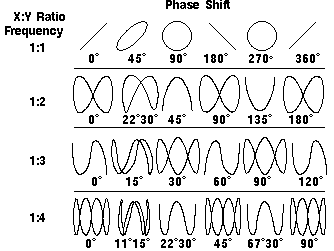Home > Electronic Tutorials > Oscilloscope Tutorial > Phase Shift Measurements

Online Oscilloscope Tutorial

# About OSCILLOSCOPE Phase Shift Measurements

## Phase Shift Measurements in Oscilloscope

The horizontal control section may have an XY mode that lets you display an input signal rather than the time base on the horizontal axis. (On some digital oscilloscopes this is a display mode setting.) This mode of operation opens up a whole new area of phase shift measurement techniques.

The phase of a wave is the amount of time that passes from the beginning of a cycle to the beginning of the next cycle, measured in degrees. Phase shift describes the difference in timing between two otherwise identical periodic signals.

One method for measuring phase shift is to use XY mode. This involves inputting one signal into the vertical system as usual and then another signal into the horizontal system. (This method only works if both signals are sine waves.) This set up is called an XY measurement because both the X and Y axis are tracing voltages. The waveform resulting from this arrangement is called a Lissajous pattern (named for French physicist Jules Antoine Lissajous and pronounced LEE-sa-zhoo). From the shape of the Lissajous pattern, you can tell the phase difference between the two signals. You can also tell their frequency ratio. Figure  shows below Lissajous patterns for various frequency ratios and phase shifts.Lissajous Patterns